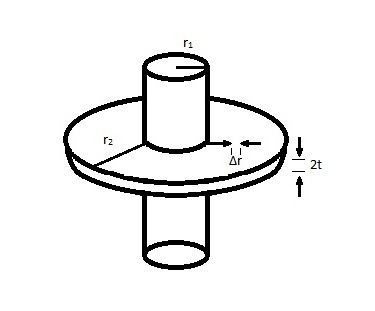# Annular fin

Updated on
Covid-19In thermal engineering, an annular fin is a specific type of fin used in heat transfer that varies, radially, in cross-sectional area. Adding an annular fin to an object increases the amount of surface area in contact with the surrounding fluid, which increases the convective heat transfer between the object and surrounding fluid. Because surface area increases as length from the object increases, an annular fin transfers more heat than a similar pin fin at any given length. Annular fins are often used to increase the heat exchange in liquid–gas heat exchanger systems.

## Governing Equation

To derive the governing equation of an annular fin, certain assumptions must be made. The fin must have constant thermal conductivity and other material properties, there must be no internal heat generation, there must be only one-dimensional conduction, and the fin must be at steady state.

Applying the energy conservation principle to a differential element between radii r and r + Δr yields

q ( 2 π r ) ( 2 t ) | r q ( 2 π r ) ( 2 t ) | r + Δ r h c ( 2 ) 2 π r Δ r ( T T e ) = 0 ,

where the first two terms are heat transferred through conduction, while the third is heat lost due to convection with the surrounding fluid. T represents the temperature at r and Te represents the temperature of the surrounding fluid. Next, applying Fourier's law

q = k T r

and dividing by 4πΔr, letting Δr → 0, yields

r ( r T r ) h c r T T e k t = 0.

Assigning new variables z

z = r h c k t

and θ, where Tb is the temperature at the base of the fin,

θ = T T e T b T e

results in the governing equation for heat transfer of an annular fin:

z 2 2 θ z 2 + z θ z z 2 θ = 0.

## Heat loss and efficiency

The maximum possible heat loss from an annular fin occurs when the fin is isothermal. This ensures that the temperature difference between the fin and the surrounding fluid is maximized at every point along the fin, increasing heat transfer by convection, and ultimately heat loss Q:

Q = k ( 4 π r 1 t ) ( T b T e ) β [ C 2 K 1 ( β r 1 ) C 1 I 1 ( β r 1 ) ] .

The efficiency ηf of an annular fin is the ratio of its heat loss to the heat loss of a similar isothermal fin:

η f = 2 r 1 β K 1 ( β r 1 ) I 1 ( β r 2 ) I 1 ( β r 1 ) K 1 ( β r 2 ) r 2 2 r 1 2 K 0 ( β r 1 ) I 1 ( β r 2 ) + I 0 ( β r 1 ) K 1 ( β r 2 ) .

## References

Similar Topics
Shuddh Desi Romance
Massimiliano Blardone
Jim Judd
Topics